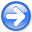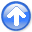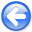Tomographic full waveform inversion and linear modeling of multiple scatteringNext: Conclusions Up: Biondi: TFWI and multiple Previous: Gradient computation with TFWI

# Numerical 1D example

I will use a simple 1D numerical example to analyze some of the characteristics of the TFWI method I presented in the previous sections. Figure 3 shows the difference between the background wavefield propagated with=1.2 km/s and the wavefield propagated with the true velocity of=1.13 km/s. The difference wavefield is displayed as a function of propagation distance and traveltime. The data are recorded with a receiver located at 7 km for a total of 4 km offset from the source. The velocity error is sufficiently high that the wavefields are completely out of phase at the receiver location. We are therefore in the situation described by equation 10. Because the events are out of phase at the receiver, the backprojection of the data residual into the velocity model yields a gradient (equation 11) that is substantially zero, as it can be verified in Figure 2. Conventional FWI would have troubles to converge even for this simple problem.

Init-Residual
Figure 3.
Difference between background wavefield computed with the starting velocity (1.2 km/s) and the wavefield propagated with the true velocity (1.13 km/s).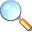Delta-Vel-Extend
Figure 4.
Extended velocity perturbation chosen to approximately model the wavefield difference shown in Figure 3 by applying equation 17.On the contrary, the linearized modeling equation defined in equation 17 would have no troubles to model the data residual. For example, we can easily reproduce the wavefield difference shown in Figure 3 by setting the extended-velocity perturbation to be a delta function along theaxis, where the shift of the delta function linearly increases with the distance from the origin. This linear shift is computed by integrating the difference in slowness between the background model and the true model. The extended-velocity perturbation is shown in Figure 4. Figure 5 shows the result of solving equation 17 with the model shown in Figure 4. The approximation of the scattered wavefield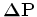is almost identical to the wavefield difference shown in Figure 3.

Next step is the backprojection of the data residual recorded by extracting the wavefields at the receiver position into the extended velocity model. In the previous section, I explained that there are three terms in this backprojection. In this case, the first and the third are zero. As discussed previously, the first component is zero because the events in the data residual are out of phase. The third term is zero because the starting extended model is zero at non-zero time lags. Figure 6 shows the contribution of the second component of the gradient. It is zero on the right of the receiver location, and it is basically constant as function of the propagation distance on the left of the receiver location.

Lin-Forw-Ext-new
Figure 5.
Perturbed wavefield computed by solving equation 17 with the model shown in Figure 4.Back-Vel-Extend
Figure 6.
Extended-velocity gradient computed by applying the adjoint of the linear operator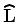to the data residuals measured at the receiver located at a distance of 7 km.Forward modeling solving equation 17 with the model shown in Figure 6 yields a scattered wavefield that at the receiver location is extremely close to the original residual shown in Figure 3. Therefore, the first term of the objective function 22 has a well-behaved parabolic shape as a function of the step size applied to the search direction, with well-defined minimum that determines the extended model after a first iteration of an iterative inversion algorithm.

The extended model obtained at the first iteration can then be used to compute the data residuals and gradient at the second iteration. Since the data residual are small, the main contribution to the gradient comes from the second term of the objective function; that is, the focusing operator. It is straightforward to verify that for the choice of focusing operator in 23, the most significant component of this gradient is away from the zero time lag. The projection of this gradient in the data space has a non-zero component and will create a data residual, which can in turn be backprojected into the velocity model. The most interesting component of this backprojection is at zero-time lag since it is the one that will effect the propagation velocity of the background wavefield at the next iteration.

Among the three gradient components discussed in the previous section, only the third one effects the extended velocity at zero-time lag. Figure 7 shows the search direction obtained by averaging this gradient component along the whole propagation interval as a function of the true velocity. As before the the starting velocity is 1.2 km/s. In contrast with the conventional FWI search direction shown in Figure 2, the search directions shown in Figure 7 is always negative for a true velocity lower than 1.2 km/s, and always positive for a true velocity larger than 1.2 km/s. This indicates that no matter how large the initial velocity error, the TFWI method will start moving the zero-lag component of the extended model in the correct direction. This result is far from being a proof of global convergence, but is definitely encouraging.Tomographic full waveform inversion and linear modeling of multiple scattering